ChemTalk

# The Schrödinger Equation## Core Concepts

In this tutorial you will learn what the Schrödinger Equation is, and how it can be used to understand the behavior of quantum systems.

## What is the Schrödinger Equation?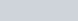The Schrödinger equation is the fundamental quantum mechanical equation which relates the wavefunction of a system to its energy. It is a linear partial differential equation which uses the operator known as the Hamiltonian to determine the energy of a system. The Hamiltonian has one term for the kinetic energy and one term for the potential energy of the system. The Hamiltonian is different for each system it is used to study. The Schrödinger Equation is extremely useful in that it can be solved for the energies and wavefunctions of a quantum system and serves as the foundation for our understanding of atoms and molecules.

## The One-Dimensional Schrödinger Equation

One of the simplest ways to use the Schrödinger equation is to determine the energy of an electron in a one-dimensional box. The electron has wavefunctions that are standing waves within the box the electron is confined to.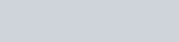V is a function describing the potential of the system. In this case, the potential is zero within the box, and infinite outside the box, guaranteeing that the electron will remain in the box. The differential equation is solved to yield a wavefunction and an energy. This differential equation also has boundary conditions for each side of the box.The one-dimensional Schrödinger equation is rarely used in practice to accurately determine the energy of a system. The model’s simplicity is helpful in understanding the energy of simple electronic systems, and how differences in energy levels are derived from nodal differences. However, the application of this Hamiltonian to even the most simple atoms renders it fairly useless due to its inaccuracy.

## Solving the Schrödinger Equation

The general solution to the Schrödinger Equation for the particle in a box is: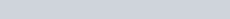Considering the aforementioned boundary conditions, we can simplify the solution further to include only the sine component.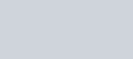## The Quantum Harmonic Oscillator Schrödinger Equation

While the one-dimensional Schrödinger equation can account for the kinetic and potential energy of an atom somewhat consistently, it does not account for the oscillatory behavior of quantum systems derived from their various wavefunctions. To account for the potential differences brought about by equilibria points we use the hamiltonian shown below.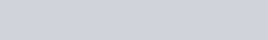This Hamiltonian results in equally spaced energy levels, unlike the one dimensional “particle in a box” model. This model is often used to described the vibrational modes of molecules. The harmonic oscillator potential describes the spring-like nature of the chemical bonds between the atoms.

## The Three-Dimensional Schrödinger Equation

While the one-dimensional Schrödinger equation may be simple to solve, many systems we wish to study exist in three dimensions. The three dimensional Schrödinger equation may be written in Cartesian coordinates or spherical coordinates, depending on which fits the system best.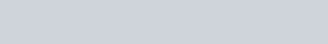However, this Hamiltonian is often simplified using a symbol called “del”. Squaring this operator means applying it twice, or taking the second partial derivative with respect to each coordinate.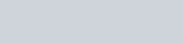## Using Spherical Polar Coordinates

Electrons in atoms are most naturally described using spherical coordinates, where the nucleus is fixed at the origin and the position of an electron is described using the radial position r, the polar angle θ, and the azimuthal angle φ.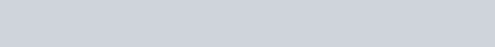## Eigenfunctions and Eigenvalues

Solving to the Schrödinger equation yields eigenvalues and eigenfunctions. These eigenfunctions are the wavefunctions of the system, and each one has a distinct eigenvalue, representing a discreet energy the system can have. A classical interpretation of the system would allow the energy to vary continuously, however numerous experiments support the quantum model, where the system may have only a discreet set of energies. Other quantum operators besides the Hamiltonian can have eigenvalues and eigenfunctions too, meaning that energy is not the only quantity that becomes quantized at the molecular and atomic level. Another example is angular momentum, which results in different shapes for s, p, d, and other atomic orbitals.

## Potential Energy

The V term in the Schrödinger equation represents potential energy. It varies depending on the system being studied. The bond in a diatomic molecule may be described using a harmonic potential energy function, while more complex molecules have several terms, accounting for electron-electron repulsion as well as electron-nucleus attraction.